Return calculation in the spotlight: Only the time-weighted return ensures comparability

The comparability of returns is the basis for any investment decision. Only if the returns of various investments and portfolios are objectively comparable, you can make a good decision. For this reason, justETF always calculates the time-weighted rate of return, thus ensuring comparability in performance measurement.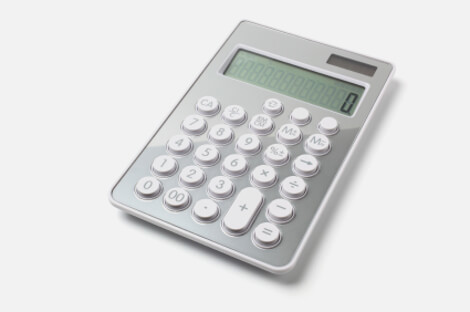justETF calculates the time-weighted return for portfolios and ETFs. This is important, if you want to compare different portfolios and individual ETFs with each other. Especially, if you make contributions on a regular basis, this should be the preferred calculation methodology for returns.

But there are also other methods to measure the performance of an investment. These include the simple return calculation and the money-weighted rate of return (also known as internal rate of return). Below we will examine the advantages and disadvantages of these three methodologies. A few calculation examples will show you the main differences.

Time-weighted rate of return

The time-weighted rate of return is independent of cash flows. This provides you objective return for each portfolio over any period. Neither the amount nor the timing of payments has an impact on this return calculation. Thus, it is suitable to compare all types of investment products and investment strategies with each other. This allows you to directly compare the time-weighted return of any individual ETF with the time-weighted return of a total portfolio.

The time-weighted rate of return is the preferred industry standard and is therefore widely used by investment professionals.

Mathematically, the time-weighted return links geometrically the daily return factors.

Money-weighted rate of return

In contrast to the time-weighted rate of return, the money-weighted rate of return takes into account the timing and the amount of contributions and withdrawals. When you put money into an investment, in which the investment manager controls the cash inflows and outflows, the money-weighted return is the preferred performance calculation method. Private equity funds or closed-end funds can be mentioned as examples for this. You would also choose the money-weighted rate of return if you do market-timing in order to measure your success.

If you or your investment manager do not actively pursue market-timing, then the money-weighted rate of return over- or underestimates the performance and does not provide a meaningful return to compare your investment success with other investments.

Simple rate of return

The easiest way to calculate the return on an investment is to divide the gain or loss of an investment by the amount invested. This calculation is simple and works perfectly for investments without contributions or withdrawals. In all other cases, however, the simple return is not a good indicator for the growth of your portfolio.

Now let's compare the return calculation methods in a few case studies to understand the differences.

If you invest 5,000 £ in an ETF portfolio and after one year these are 5,500 £, then you have earned a return of 10%. If you're investing for another year and the portfolio increases by +10% in the second year, then your overall return is 21%. Since there were no contributions or withdrawals within the whole period, all three calculation methods provide the same result.

Return calculation methods for an investment of 5,000 £ in comparisonCase Study 1: Initial investment of 5,000 £ / Final value of 6,050 £ after two years / Performance of +10% in the first year and +10% in the second year

Calculation

What does it mean, if you contribute another 5,000 £ after one year into this strategy? The performance according to the simple return calculation is now 15.5%. This would mean your investment has underperformed, only because you have contributed additional money after one year. Of course, this is not the case. In this case, the simple return calculation is not suitable.

Because the performance in both years is equal, the money-weighted and time-weighted returns provide the same result. The timing of the contribution does not have any effect.

The influence of a contribution of 5,000 £ on the different return calculation methods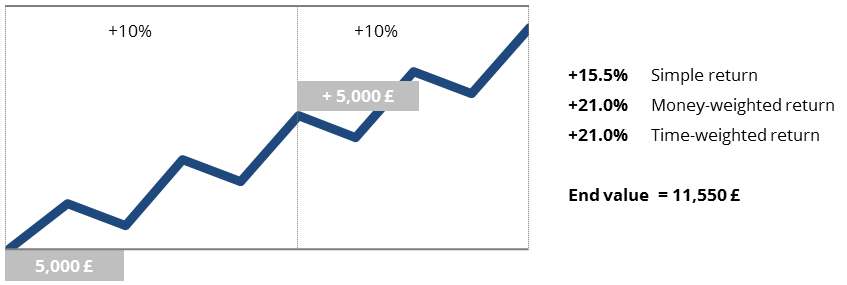Case Study 2: Initial investment of 5,000 £ / Contribution of 5,000 £ after one year / Final value of 11,550 £ after two years / Performance of +10% in the first year and +10% in the second year

Calculation

The picture changes, when your portfolio has developed differently in the second year. Suppose, in the first year your portfolio has grown by + 35% and in the second year it has only generated + 20%. If you do not contribute or withdraw any money then all calculation methods again provide the same result.

Return calculation methods in comparison over two periods with differing returns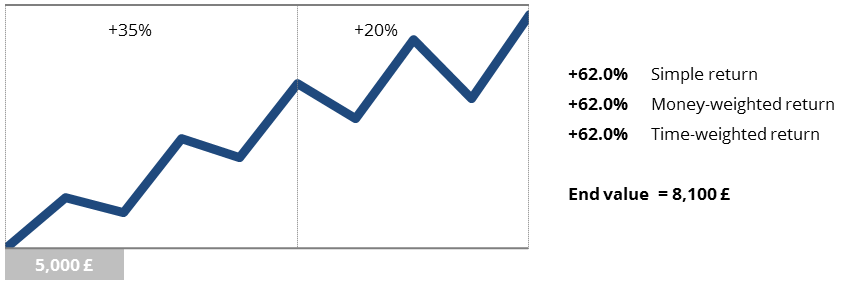Case Study 3: Initial investment of 5,000 £ / Final value of 8,100 £ after two years / Performance of +35% in the first year and +20% in the second year

Calculation

The case study illustrates the difference between the money-weighted and time-weighted rate of return. It does make a difference when you contribute the money into your strategy if the performance is different in each year. In retrospect, it would of course have been advantageous to directly contribute all money into the portfolio in the first year, since the increase in value was higher than in the second year. For this reason, the money-weighted return results in a lower return of 56.8% over two years. If you want to compare the performance of your portfolio with alternative strategies or single ETFs on the FTSE 100 or MSCI World, you can only do this by comparing their time-weighted returns.

The influence of a contribution of 5,000 £ on the different return calculation methods for two periods with differing returnsCase Study 4: Initial investment of 5,000 £ / Contribution of 5,000 £ after one year / Final value of 14,100 £ after two years / Performance of +35% in the first year and +20% in the second year

Calculation

The calculation methodology may also have an influence on the sign of the calculated return. Thus it is possible that, for example, the time-weighted return provides a positive result, while the money-weighted or simple return results in negative rates of return.

This fact holds considerable potential for confusion. However, if you want to measure the success of your portfolio with alternative strategies or a benchmark (such as the FTSE 100), you need to take the time-weighted return of 8.0% to draw the right conclusion. If the FTSE 100 would have lost -5.0% in the same period, you would not have been able to draw the right conclusion by comparing the simple or money-weighted returns in this case study. Your investment strategy has developed much better with 8.0% as compared to -5.0%.

The influence of a negative development in the second year on the different return calculation methods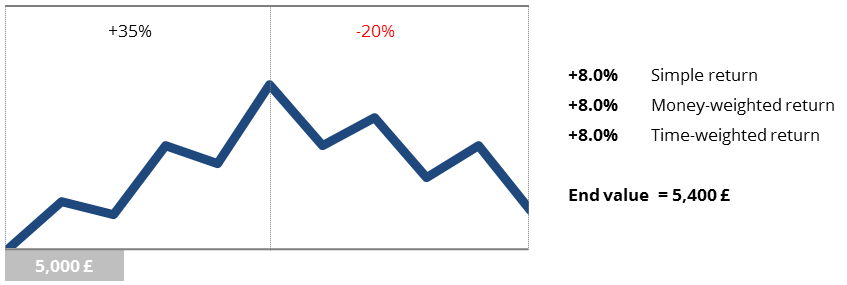Case Study 5: Initial investment of 5,000 £ / Final value of 5,400 £ after two years / Performance of +35% in the first year and -20% in the second year

Calculation

The influence of a contribution of 5,000 £ on the different return calculation methods for a negative development in the second year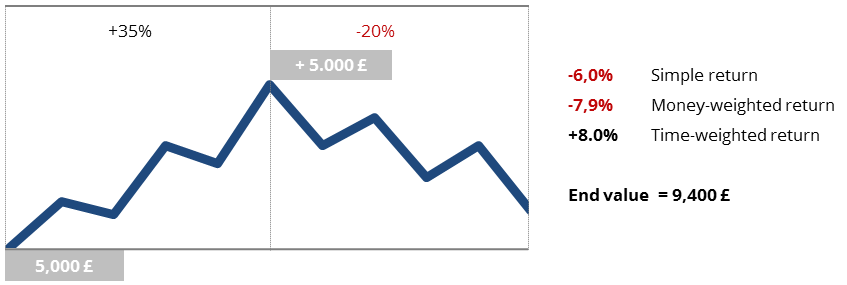Case Study 6: Initial investment of 5,000 £ / Contribution of 5,000 £ after one year / Final value of 9,400 £ after two years / Performance of +35% in the first year and -20% in the second year

Calculation